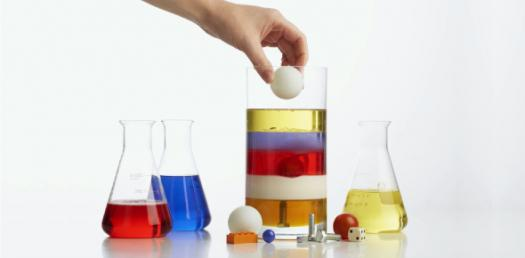# Can You Find Out The Density? Trivia Questions Quiz

5 Questions | Attempts: 523
ShareSettings.

• 1.
Three objects are cut from the same sheet of steel. They are different shapes but they all have the same mass.                   disc                                          square                                   L-shape Which object has the greatest density?
• A.

All have the same density

• B.

The disc

• C.

The square

• D.

The L-shape

• E.

None of the above is the answer

• 2.
The mass of a paper clip is 0.50grams and the density of its material is 8.0g/cm3. The total volume of the number of clips is 20cm3. How many paper clips are there
• A.

320

• B.

160

• C.

240

• D.

80

• E.

10

• 3.
The level of water in a measuring cylinder is 75cm3. A stone of volume 20cm3 is lowered into the water. What is the new reading of the water level?
• A.

95cm3

• B.

55cm3

• C.

75cm3

• D.

20cm3

• 4.
If a block of copper measures 2.00cm  x  4.00cm  x  5.00cm and weighs 356grams. What is it density?
• A.

8.9g/cm

• B.

8.9kg/m3

• C.

8900kg/m3

• D.

8900g/cm

• E.

None of the above is the answer

• 5.
Bonus Questions! A box has an internal volume of 1000cm3. When a solid object is placed in a closed box, the volume of air in the box is 520cm3. The density of the object is 8.00g/cm3. What is the mass of the object? The mass of the object is ________________grams. (Enter your answer in the box)

## Related TopicsBack to top
×

Wait!
Here's an interesting quiz for you.